# Qubit Placement

This notebooks walks through qubit placement runtime features exposed through the `cirq_google.workflow` tools.Note: this notebook relies on unreleased Cirq features. If you want to try these features, make sure you install cirq via `pip install cirq --pre`.

``````try:
import cirq
except ImportError:
print("installing cirq...")
# This depends on unreleased (as of 1.14) qubit placement functions.
!pip install --quiet cirq --pre
print("installed cirq.")
import cirq
``````
``````import numpy as np
from matplotlib import pyplot as plt
``````

## Target Device

First, we get an example target device and---crucially---its qubit connectivity graph.

``````from cirq_google.workflow import SimulatedProcessorWithLocalDeviceRecord
rainbow_record = SimulatedProcessorWithLocalDeviceRecord('rainbow')
rainbow_device = rainbow_record.get_device()

_ = cirq.draw_gridlike(rainbow_graph, tilted=False)
``````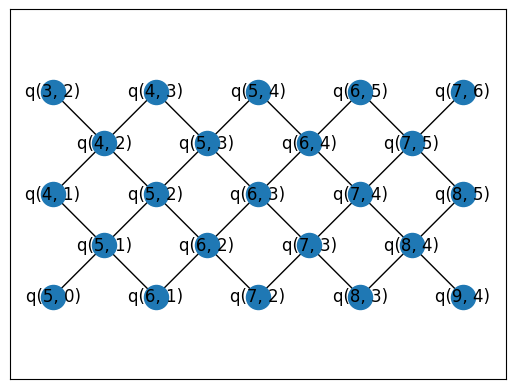## Target problem topology

We'll use a `NamedTopology` to talk about the graph connectivity of our circuit. In this case, we'll construct a random circuit on a `cirq.TiltedSquareLattice` topology of a given width and height.

``````topo = cirq.TiltedSquareLattice(3, 2)
_ = cirq.draw_gridlike(topo.graph, tilted=False)
``````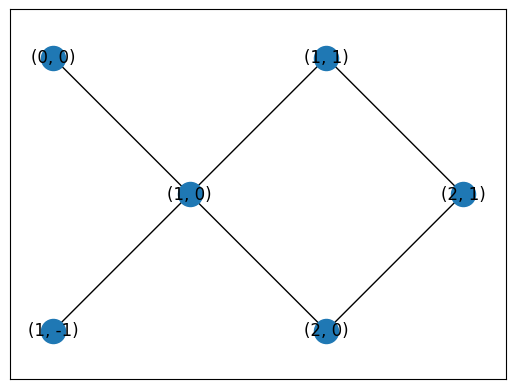``````circuit = cirq.experiments.random_rotations_between_grid_interaction_layers_circuit(
qubits=sorted(topo.nodes_as_gridqubits()),
depth=4,
)

from cirq.contrib.svg import SVGCircuit
SVGCircuit(circuit)
``````
```findfont: Font family 'Arial' not found.
```### Verify circuit connectivity

We use a topology to generate a random circuit. Now we can extract the implied circuit topology from the two qubit gates within to verify that it is indeed the topology we requested:

``````from cirq.contrib.routing import get_circuit_connectivity
circuit_graph = get_circuit_connectivity(circuit)
_ = cirq.draw_gridlike(circuit_graph, tilted=False)
``````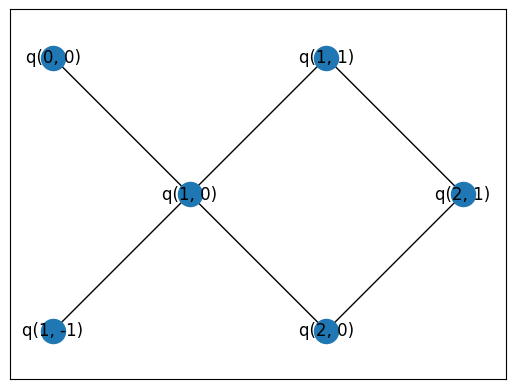## QubitPlacer

The following classes follow the `QubitPlacer` interface. In particular, there is a method `place_circuit` which maps arbitary input qubits in a circuit to qubits that exist on the device. It accepts a named problem topology and other runtime information to provide more context to the qubit placers.

``````# set up some other required arguments.
# In a real `cirq_google.workflow.execute()` run, these will be
# handled for you.

shared_rt_info = SharedRuntimeInfo(run_id='example', device=rainbow_device)

rs = np.random.RandomState(10)
``````

## RandomDevicePlacer

The `RandomDevicePlacer` will find random, valid placements. On a technical level, this uses networkx subgraph monomorphism routines to map the problem topology to the device graph.

``````from cirq_google.workflow import NaiveQubitPlacer, RandomDevicePlacer, HardcodedQubitPlacer
``````
``````rdp = RandomDevicePlacer()

placed_c, placement = rdp.place_circuit(circuit, problem_topology=topo, shared_rt_info=shared_rt_info, rs=rs)
cirq.draw_placements(rainbow_graph, circuit_graph, [placement])
``````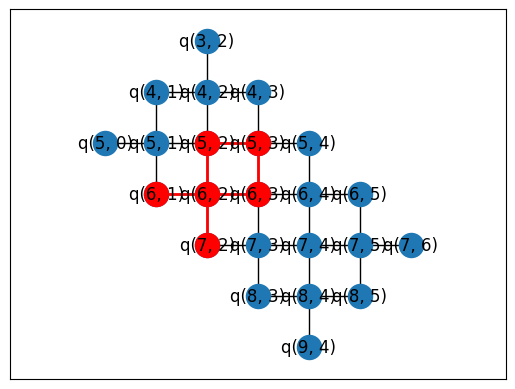## NaiveQubitPlacer

As a fallback, you can rely on `NaiveQubitPlacer` which will map input qubits to output qubits. Be careful though! This means you have to choose your qubits as part of circuit construction, which is not advised if you're using `cirq_google.workflow` best practices.

``````naive = NaiveQubitPlacer()

placed_c, placement = naive.place_circuit(circuit, problem_topology=topo, shared_rt_info=shared_rt_info, rs=rs)
cirq.draw_placements(rainbow_graph, circuit_graph, [placement])
``````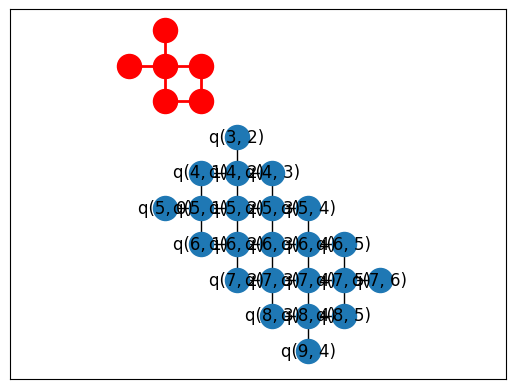## HardcodedQubitPlacer

If you want ultimate control over qubit placement but still want to decouple your `cg.QuantumExecutable`s from a particular device or configuration, you can use the `HardcodedQubitPlacer` to place your circuits at runtime but from a pre-specified list of valid placements.

Here, we introduce a helper function to generate placements for all `TiltedSquareLattice` topologies anchored from qubit `(4, 2)`.

``````import itertools

def all_offset_placements(device_graph, offset=(4, 2), min_sidelength=2, max_sidelength=5):
# Generate candidate tilted square lattice topologies
sidelens = list(itertools.product(range(min_sidelength, max_sidelength + 1), repeat=2))
topos = [cirq.TiltedSquareLattice(width, height) for width, height in sidelens]

# Make placements using TiltedSquareLattice.nodes_to_gridqubits offset parameter
placements = {topo: topo.nodes_to_gridqubits(offset=offset) for topo in topos}

# Only allow placements that are valid on the device graph
placements = {topo: mapping for topo, mapping in placements.items()
if cirq.is_valid_placement(device_graph, topo.graph, mapping)}
return placements
``````

The constructor for `HardcodedQubitPlacer` takes in a mapping from named topology to a "placement". Each placement is a mapping from named topology node to device qubit.

``````rainbow_mapping = all_offset_placements(rainbow_graph)
hardcoded = HardcodedQubitPlacer(mapping=rainbow_mapping)

placed_c, placement = hardcoded.place_circuit(circuit, problem_topology=topo, shared_rt_info=shared_rt_info, rs=rs)
cirq.draw_placements(rainbow_graph, circuit_graph, [placement])
``````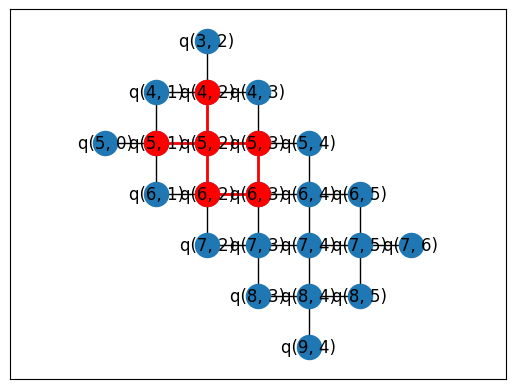#### All hardcoded placements

For completeness, the following figure shows all hardcoded placements. If you request one of the supported `TiltedSquareLattice` topology, you'll get the depicted mapping. If you request a topology not in the hardcoded list, you will receive an error. The `RandomDevicePlacer` (in contrast) will always succeed if the topology can be placed on the device.

``````from math import ceil

n_col = 3
n_row = int(ceil(len(rainbow_mapping)/n_col))
fig, axes = plt.subplots(n_row, n_col, figsize=(4*n_col, 3*n_row))
axes = axes.reshape(-1)
for i, (topo, mapping) in enumerate(rainbow_mapping.items()):

axes[i].set_title(f'{topo.width}x{topo.height}')
cirq.draw_placements(rainbow_graph, topo.graph, [mapping],
tilted=False, axes=axes[i:i+1])

fig.suptitle("All hardcoded placements", fontsize=14)
fig.tight_layout()
``````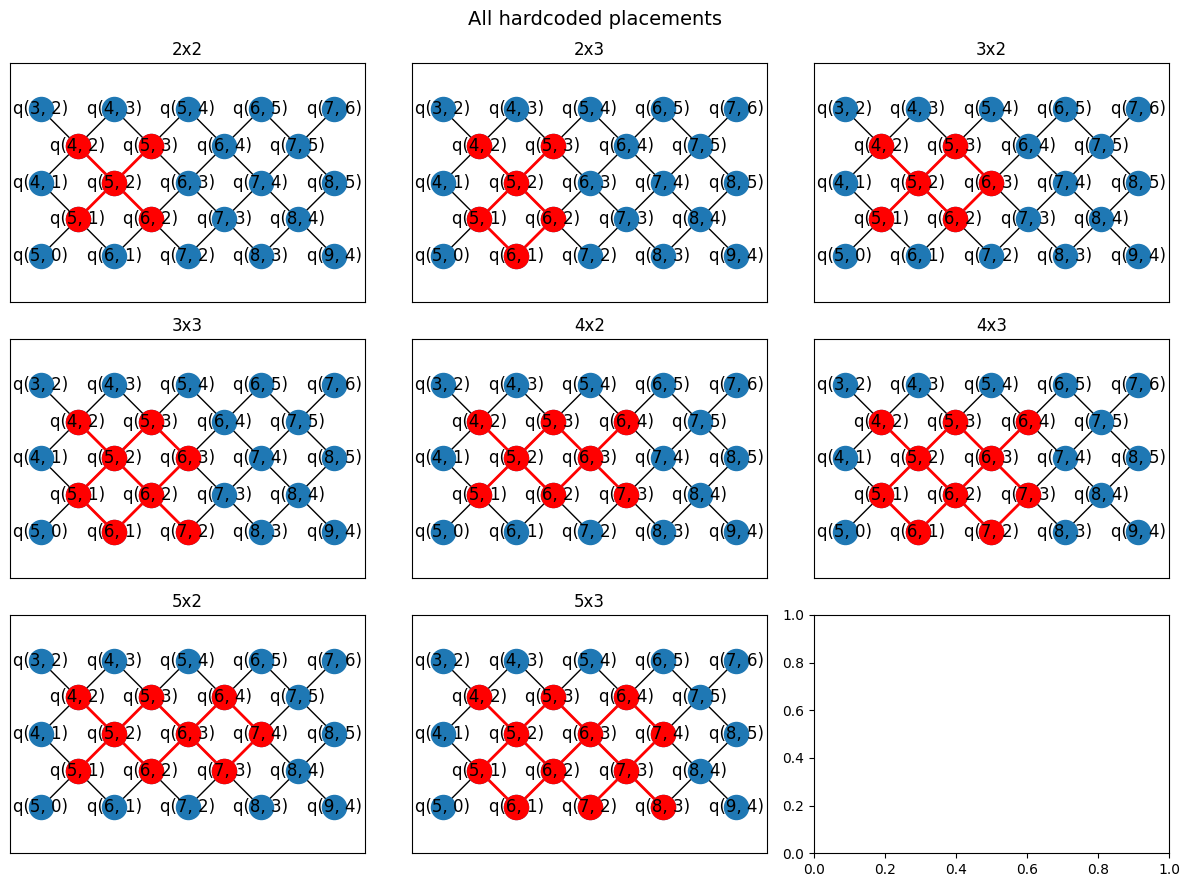[{ "type": "thumb-down", "id": "missingTheInformationINeed", "label":"Missing the information I need" },{ "type": "thumb-down", "id": "tooComplicatedTooManySteps", "label":"Too complicated / too many steps" },{ "type": "thumb-down", "id": "outOfDate", "label":"Out of date" },{ "type": "thumb-down", "id": "samplesCodeIssue", "label":"Samples / code issue" },{ "type": "thumb-down", "id": "otherDown", "label":"Other" }]
[{ "type": "thumb-up", "id": "easyToUnderstand", "label":"Easy to understand" },{ "type": "thumb-up", "id": "solvedMyProblem", "label":"Solved my problem" },{ "type": "thumb-up", "id": "otherUp", "label":"Other" }]